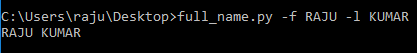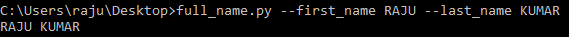Related Articles

# Getopt module in Python

• Last Updated : 04 May, 2020

The getopt module is a parser for command-line options based on the convention established by the Unix `getopt()` function. It is in general used for parsing an argument sequence such as sys.argv. In other words, this module helps scripts to parse command-line arguments in sys.argv. It works similar to the C `getopt()` function for parsing command-line parameters.

## Python getopt function

The first function provided by this module is of the same name i.e. `getopt()`. Its primary functionality is to parse command-line options and parameter list. The syntax of the function is as below:

Syntax: getopt.getopt(args, options, [long_options])

Parameters:
args: List of arguments to be passed.
options: String of option letters that the script wants to recognize. Options that require an argument should be followed by a colon (:).
long_options: List of the string with the name of long options. Options that require arguments should be followed by an equal sign (=).

Return Type: Returns value consisting of two elements: the first is a list of (option, value) pairs. The second is the list of program arguments left after the option list was stripped.

Example 1:

 `import` `sys``import` `getopt`` ` ` ` `def` `full_name():``    ``first_name ``=` `None``    ``last_name ``=` `None`` ` `    ``argv ``=` `sys.argv[``1``:]`` ` `    ``try``:``        ``opts, args ``=` `getopt.getopt(argv, ``"f:l:"``)``     ` `    ``except``:``        ``print``(``"Error"``)`` ` `    ``for` `opt, arg ``in` `opts:``        ``if` `opt ``in` `[``'-f'``]:``            ``first_name ``=` `arg``        ``elif` `opt ``in` `[``'-l'``]:``            ``last_name ``=` `arg``     ` ` ` `    ``print``( first_name ``+``" "` `+` `last_name)`` ` `full_name()    `

Output:Here we have created a function `full_name()`, which prints the full name after getting first name and last name from the command line. We have also abbreviated first name as ‘f’ and last name as ‘l’.

Example 2: Now lets look into the case where instead of short-form like ‘f’ or ‘l’, we could use full forms as ‘first_name’ and ‘last_name’.The below code uses full forms to print full name;

 `import` `sys``import` `getopt`` ` `def` `full_name():``    ``first_name ``=` `None``    ``last_name ``=` `None`` ` `    ``argv ``=` `sys.argv[``1``:]`` ` `    ``try``:``        ``opts, args ``=` `getopt.getopt(argv, ``"f:l:"``, ``                                   ``[``"first_name ="``,``                                    ``"last_name ="``])``     ` `    ``except``:``        ``print``(``"Error"``)`` ` `    ``for` `opt, arg ``in` `opts:``        ``if` `opt ``in` `[``'-f'``, ``'--first_name'``]:``            ``first_name ``=` `arg``        ``elif` `opt ``in` `[``'-l'``, ``'--last_name'``]:``            ``last_name ``=` `arg``     ` ` ` `    ``print``( first_name ``+``" "` `+` `last_name)`` ` `full_name()`

Output:Note: The single dash(‘-‘) for short forms and double dash(‘–‘) for long form of the argument in the code should be used.

Attention geek! Strengthen your foundations with the Python Programming Foundation Course and learn the basics.

To begin with, your interview preparations Enhance your Data Structures concepts with the Python DS Course. And to begin with your Machine Learning Journey, join the Machine Learning – Basic Level Course

My Personal Notes arrow_drop_up